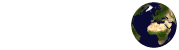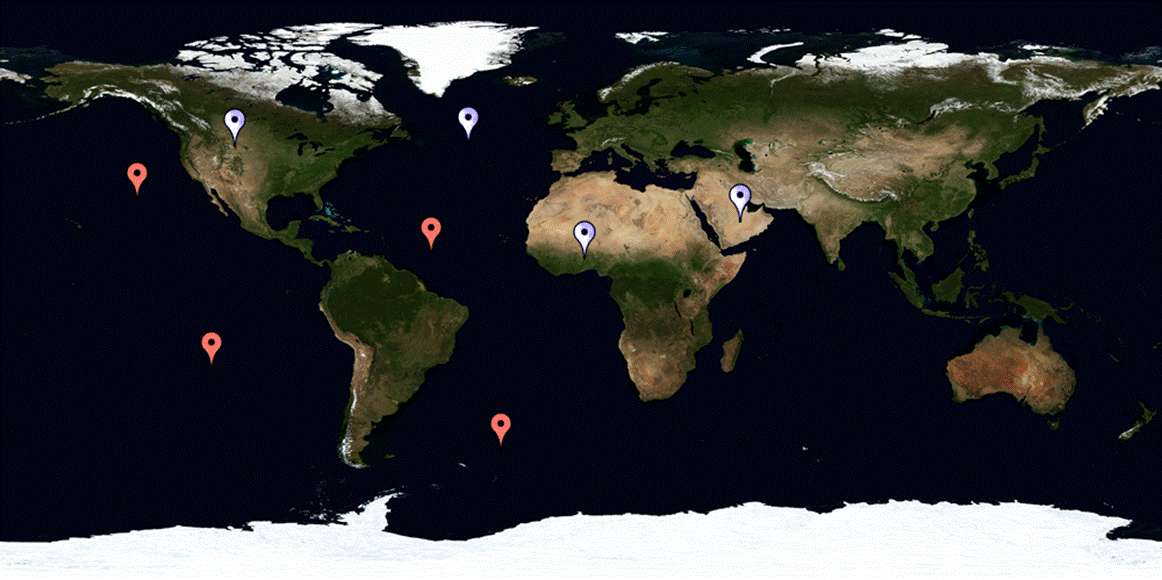Introduction

The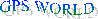project is the first AtMega32 based GPS receiver ( back end) that can track satellites and calculate the navigation solution from raw digital data.

The navigation solution is the most important goal of GPS design from the view point of the user. It is calculated by using the observables in a process that involves solving non-linear equations trough the use of Newton-Raphson technique (later discussed in this text). Such a navigation solution can be calculated by slow microprocessors, or even by feeding RINEX2 data) and feeding it to a computer with a program such as MATLAB. In the Flex Laboratory at Cornell University the GPS receiver used is based on a Real Time Linux PCI solution built by Aerospace Innovations.  Essentially what Aerospace Innovations accomplish was to implement a GPS receiver where the PC could take the place of the microprocessor. In Figure 1 is shown a similar design developed by Zarlink which employs the ARM60 32 bit RISC processor to calculate the navigation solution while in Figure 2 is shown the schematic of what Aerospace Innovation accomplished with the Real Time Linux PCI solution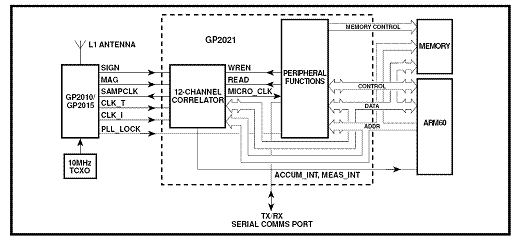Figure 1. Structure of a GPS receiver by Zarlink  using the ARM60 32 bit RISC processor.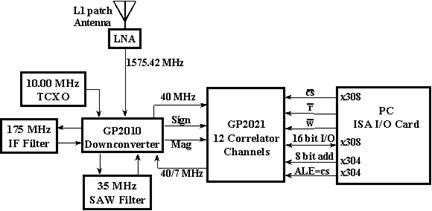Figure 2.Structure of a GPS receiver using PC ISA I/O Card.

In the  figures above one can see three main parts: The RF Section (GP 2010/2015) , the Correlator Section (GP 2021), and either the ARM60 Risc Processor (the microcrontroller) or the PC interface through either ISA or PCI solutions to implement the Control Section where the navigation solution is implemented.  In the following sections we will deal with the implementation of the Control Section in the Atmega32 microcontroller.Top

High Level Design

Rationale:

The GPS WORLD project was inspired in our desire to combine our experience in microcontroller design with our knowledge of GPS theory. Prof. Paul Kintner offered to lend us a very good receiver (DG14) produced by THALES that implements the RF Section, and the Correlator Section. The DG14 is also capable of outputting the raw digital data (from the Correlator Section or GP 2021 chip) through the RS-232 serial communication interface. In past ECE 476 GPS related projects the technology was used to develop many useful and insightful applications, but the fact that through the many years of the course no one had really implemented the technology itself also inspired us to be the first to do it. This of course raised many questions about the capabilities of the Atmega32 microcontroller and how realistic our goal was since we knew the project required high degrees of accuracy and intensive calculations. In the next sections will we show our ups and downs that left to many design decisions which at the end of the day got us a fully functional back end of a receiver.

Background math:

Coordinate transformation:

Once we have calculated the position of the satellites and our own we need to out put it in a more comprehensible format. Recall that the calculation were carried out using Cartesian Earth-Centered-Earth-Fixed (ECEF) X, Y, Z coordinates but the official reference frame of GPS is the World Geodetic System 1984 (WGS-84) latitude (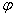), longitude (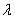), and altitude(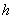). This section explains the transformations which our project undertakes when representing the GPS results in ellipsoidal coordinates from Cartesian coordinates.

Given the (X, Y, Z)ECEF the longitude (in degrees) may be calculated using the following equation: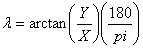. For the other two components an iterative approach is necessary since the latitude and altitude values depend on each other.  First calculate the radius of a parallel: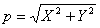, and approximate an initial latitude: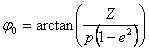, where e2 = 0.00669437998863492 is the square of the Earth’s orbital eccentricity.

1. Compute an approximate radius of curvature: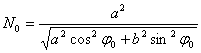,

where a = 6378137.00000 m and b = 6356752.31425 m are  the semi-major and semi-minor axis of the reference ellipsoid respectively

2. Compute the height: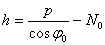3. Compute the new latitude:4.  If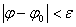(where epsilon is a very small number) then the loop ends otherwise set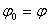and repeat step 1 through 3.

For more on coordinate transformation of GPS results please see Chapter 10 of GPS: Theory and Practice by B. Hofmann-Wellenhof. To see the algorithm in C refer to the latlong()  function in gpc.c

Newton-Raphson Method:

The Newton-Raphson method is an interactive technique for finding roots of a nonlinear function that are well behaved (their derivatives can be obtained easily). During the process of obtaining the navigation solution this method will be used, but for now we will show only the mathematical theory that in a future section will allow better understanding of the code implemented. If you desired to look at the one dimensional case you can look it up in any Calculus book, but we will jump right in to the multidimensional case since is the one that applies to the navigation solution.  The Newton-Raphson method is based on the Taylor Series expansion and for the case of three variable function such Taylor Series can be written in the form: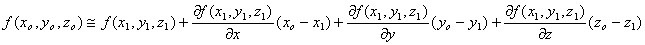where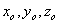are the roots of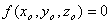and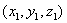are the guesses at the root. Our whole goal in this case is to obtain a solution for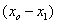,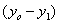,and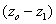.  Since,,and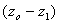are essentially three variables( say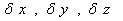respectively) in order to solve for theme we need three independent equations: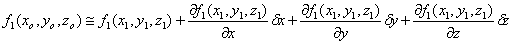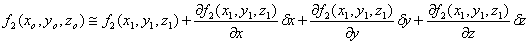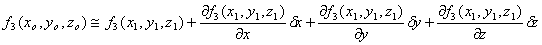The hardest part theoretically is finding the independent equations since after that the problem can be solved with some basic linear algebra. For example say :,, and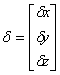then we can rewrite our system of equations in the from: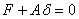which has the solution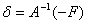.  The equations are then iterated using the new guesses,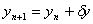,and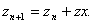To see the algorithm in C refer to the solvePos()  function in gpc.c.

Gauss-Jordan Elimination:

Solving a system of linear equations using MATLAB or even by hand is one thing and with a microcontroller is another.  When it came to solvingfor a system of four equations and four unknowns our design choice was the Gauss-Jordan elimination method. Most academics are very likely to suggest not to use Gauss-Jordan elimination method and instead to go with a variant like LU decomposition. There are good reasons why you might not want to use Gauss-Jordan elimination since it requires all the right-hand sides to be stored and manipulated at the same time, and is significantly slower than LU decomposition. Nevertheless the Gauss-Jordan method is very straightforward, and  easily to understand, and  for these reasons we chose to implement it in this version of the project .We recommend using another linear-equation solver in further developments of the project. That being set lets look over the mathematics.

Say you want to solve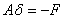: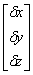=-Compose an augmented matrix with the right hand side of the equation aboveNow, perform elementary row operations to put the augmented matrix into the upper triangular formSolve the equation of the kth row for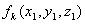(for the three dimensional case k=3 at the beginning), then substitute back into the equation of the k-1th row to obtain the solution for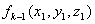an continue until you solve for all. This can be written mathematically as follows:To see the algorithm in C refer to the solvePos()  function in gpc.c

Orbital Dynamics:

In this section we discuss the orbits of GPS satellites and a very high level explanation of what the code in function FindSat() in gp.c accomplishes. If the reader desires a deeper explanation here we take the opportunity to refer him to a good physics book that explains the gravitational attraction between two points with given masses and to GPS: Theory and Practice by B. Hofmann-Wellenhof.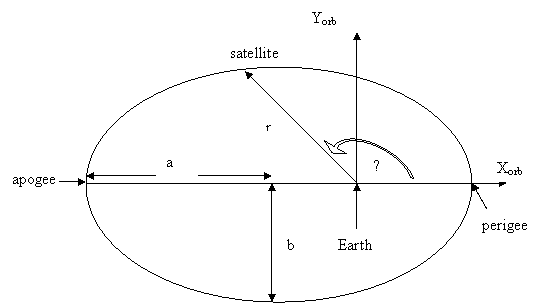Figure 3 An elliptical orbit in the orbital coordinate frame(x0,y0).

This is the shape of a satellite orbit and could be also described by equation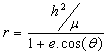where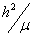is a constant for a specific orbit (not discussed here) and e is the orbital eccentricity (if e < 1 then the orbit is elliptical, if e = 1 then the orbit is circular). It turns out that there is a relation between the parameters a, e and: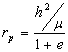,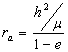,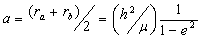,

meaning we can figure out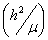given a and e or vice versa. Hence we only need two parameters to determine the shape of the orbit. We will use a  and e (which are  two of the required six ephemerides in order to know the satellites position) in FindSat() function in  gps.c .

Once we know the shape of the satellite then it is necessary to orient it in space as shown in Figure 4.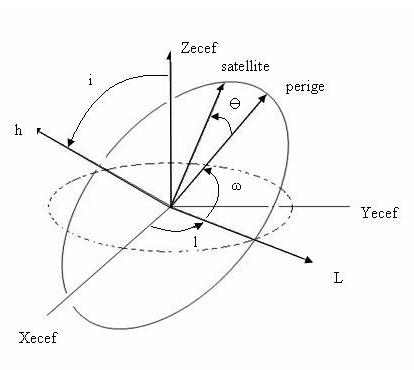Figure 4.Orbital parameters specifying a satellite position.

The extra parameters needed to orient the satellite are the inclination (i, usually around 55 degrees for all satellites), the longitude of ascending node (l), and the argument of perigee (ω).The final parameter necessary to calculate the position of the satellite is the true anomaly Ө. If we know the true anomaly we can find the satellite and no more calculations are necessary. Of course it is not that simple because the true anomaly is sent every four hours with the rest of the ephemerides implying we can only calculate the exact position for each satellite every four hours. This is not what we want but we will leave this discussion of how to calculate Ө and orbital time dependence for a future section in this text(Program/Hardware Design). Nevertheless there is a step that even though we are not going to explain in detail should be mention (carefully explained in GPS: Theory and Practice by B. Hofmann-Wellenhof). Once we use the ephemerides we know the orientation of the orbital plane with respect to the ECEF coordinate. This is grea,t but what we really want is the satellite position with respect to earth and not its own orbital plane (Xorb ,Yorb, and Zorb) as shown in Figure 3. Therefore we do some simple transformation, rotating about the needed axis to get the ECEF coordinates for each satellite. This can all be seen in the last lines of FindSat() procedure in gps.c. The complete transformation consists of multiplying three rotational matrixes:where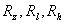are rotation matrices and refer to the axis labeled in Figure 4. Meaning we first rotate around h, then l, and finally z axis (notice that the order of the rotations affects the result).

Logical Structure: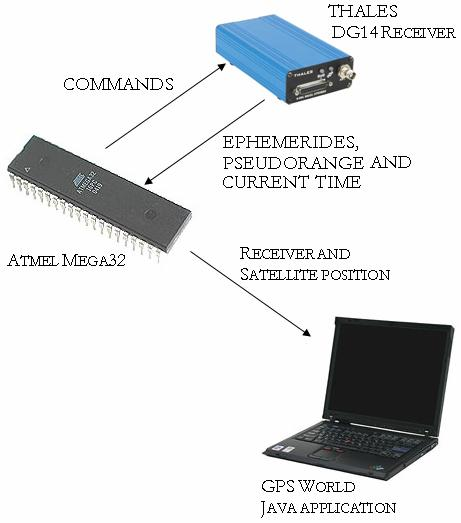Figure 5:  High-level design structure

Instruments: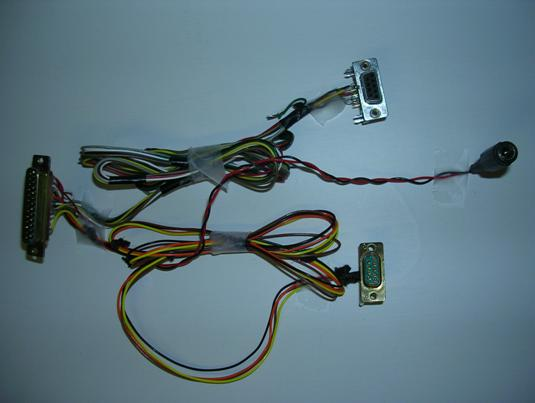Figure 6: RS 232 serial converter...Figure 7: ACC GPS antenna, model GPS-P1MAM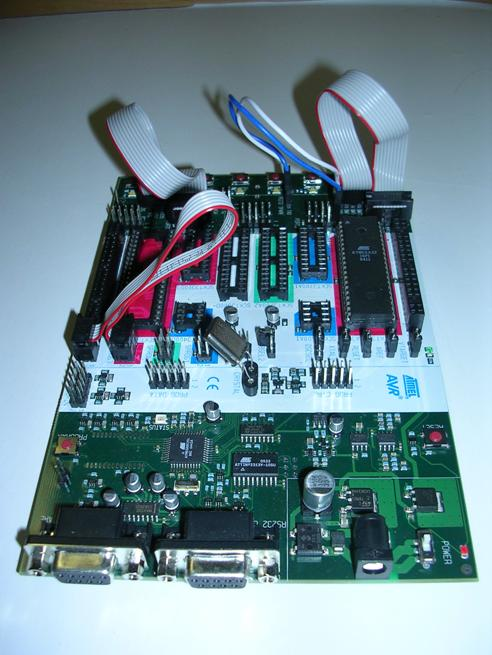Figure 8: STK500 with ATMega32 microprocessor.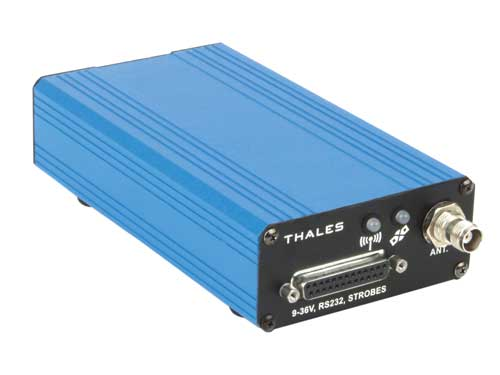Figure 9: THALES DG14 receiverTop

Program/Hardware Design

Extracting Raw Satellite Data:

The orbital ephemerides are calculated very accurately as part of the GPS control segment up-linked to the spacecraft about once per day and updated in the navigation message about every 4 hours. To convey these parameters to the user, they are encoded on the GPS C/A (civilian access) signal so that each satellite transmits its own orbital parameters. GPS receivers like the THALES DG14, acquire and store these parameters to calculate their own location and that of the satellites which they are tracking. To obtain raw ephemerid data the MCU sends the appropriate SNV command through Port B using the GetEphem() function. This function makes the receiver execute the following command: \$PASHQ,SNV,A. This command queries for ephemeris data from each satellite currently being tracked by the receiver, and outputs the response through Port A. The SNV message response has the following format: \$PASHQ,SNV,<Ephemeris data string + checksum>. The ParseEphem() function then takes care of breaking down that response message whose structure is summarized in table below. Note that the first 11 characters in this message (\$PASHQ, SNV,) are discarded by the parser.

Table 1: SNV Response message structure

 Field Bytes Content short wn 2 GPS week number long tow 4 Seconds of GPS week float tgd 4 Group delay (seconds) long aodc 4 Clock data issue long toc 4 Clock data reference time in seconds float af2 4 Satellite clock correction (s/s2) float af1 4 Satellite clock correction (s/s) float af0 4 Satellite clock correction (s) long aode 4 Orbit data issue float deltan 4 Mean anomaly correction (semicircles/s) double m0 8 Mean anomaly at reference time (semicircles) double e 8 Eccentricity double roota 8 Square root of semi-major axis (m1/2) long toe 4 Reference time for orbit or time of epoch (s) float cic 4 Inclination cosine harmonic correction (rad) float crc 4 Orbit radius cosine harmonic correction (m) float cis 4 Inclination sine harmonic correction (rad) float crs 4 Orbit radius sine harmonic correction (m) float cuc 4 Latitude cosine harmonic correction (rad) float cus 4 Latitude sine harmonic correction (rad) double omega0 8 Longitude of ascending node (semicircles) double omega 8 Argument of perigee (semicircles) double i0 8 Inclination angle (semicircles) float omegadot 4 Rate of ascension (semicircles/s) float idot 4 Rate of inclination (semicircles/s) short accuracy 2 User range accuracy short health 2 Satellite health short fit 2 Curve fit interval char PRN 1 Satellite PRN number minus 1 (0 to 31) char res 1 Reserved character checksum 2 ---

Table 2: XYZ Data String

 Field Bytes Content long[RcvTime] 4 Time at which the signal was received in milliseconds of the week referred to GPS system time. short [Total Satellites] 2 The total number of satellites appearing in the message. short [sv_n] 2 The PRN number of the satellite (being tracked on channel n of the DG14 double [satx_n] 8 The x coordinate of the satellite being tracked on channel n of the DG14 double [saty_n] 8 The y coordinate of the satellite being tracked on channel n of the DG14 double [satz_n] 8 The z coordinate of the satellite being tracked on channel n of the DG14 double [range_n] 8 The corrected pseudo-range of the satellite referenced in [sv_n] checksum 2 ---

Double to Single Precision Float Conversion:

One of the many challenges that arouse right as we were extracting the raw satellite data was that some of the ephemeris parameters which the THALES DG14 receiver sent out were double precision floats (64 bit) while the Code Vision AVR is restricted to single precision (32 bit) floating point arithmetic. This meant that unlike the other parameters which were easily parsed using the methods of the previous subsection these ephemerides needed to be converted before we could use them. For this conversion of raw, unformatted or “binary” data we first consulted the IEEE standard for floating point arithmetic. The IEEE single precision floating point number consists of a sign bit (S), followed by eight exponent bits (E), the 32 bit word is completed by 23 fraction (F) bits (see Figure 10). The IEEE double precision floating point on the other hand consist also of a sign bit (S), but it’s followed by eleven exponent bits (E), this 64 bit word representation is completed by 52 fraction (F) bits (see Figure 11).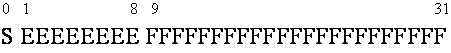Figure 10: IEEE single precision floating point representation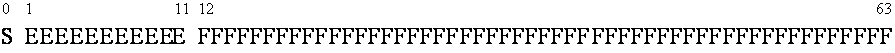Figure 11: IEEE double precision floating point representation

The ReadDouble() function takes care of the conversion which begins by parsing the 64 bit word character by character using the getchar() function in Code Vision AVR and storing those in temporary double_buffer eight element unsigned character array. Since our target is to have a 32 bit word in the end, the first element of this array (double_buffer) is casted into an unsigned long format and shifted left by 24 bits to move the original eight bits to the beginning of the word. AND’ing the result with 0b10000000000000000000000000000000 guarantees that the new word consists only of the S bit followed by 31 zeros; lets call this new word S.  Converting the exponent bits of the floating point value is a quite more complex. For the exponent section for example we first have to look into the first and second elements of the double_buffer array and shift those around so as to leave only the exponent bits in an unsigned int format (see Figure 12 below). But notice that we still have eleven exponent bits and the single precision float must have only eight. This final conversion is achieved by subtracting 1023 and adding 127 to offset the value leaving only eight 0 bits followed by eight exponent bits. This result is then casted into a 32 bit unsigned long format, and shifted left by 23 bits to set the bit in the correct position. We call this new word E.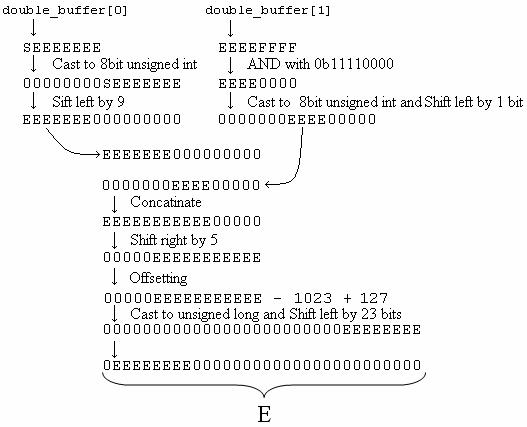Figure 12: Single precision floating point Exponent bits

To convert the fraction bits we simple took the first 23 of the original 52 bits in the double precision fraction bits. To do this we needed to look into the second, third, fourth and fifth elements of double_buffer array which as you may recall contains the raw double precision float bits. The values are assigned to temporary variables and casted into unsigned long format and later shifted left by 28, 20, 16, and 4, respectively (see Figure 13 below). These four 32 bit words are then concatenated and to guarantee that only the most significant 23 bits are used we AND the result of the concatenation with 0b11111111111111111111111000000000. Finally, to set the bits in the correct position we shift the bits in this new F word to the right by 9.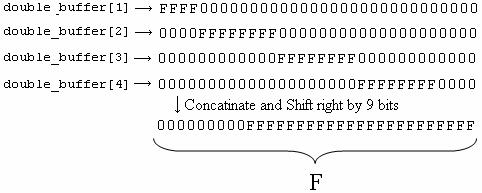Figure 13: Single precision float fraction bits

The S, E, and F words are then concatenated to obtain the single precision floating point value V.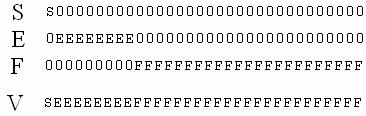Figure 14: Single precision float complete bit representation

Satellite Tracking:

Figure 15 shows a screenshot of the Java application developed by Roberto Torralbas that was used to display satellites on a world map. The program consist of tabs to also allow display of some of the raw data extracted (ephemerides, pseudoranges) and the calculated satellite coordinates as well as the navigation solution. Notice that in the screenshot there are two markers ( white ,and red) of different colors currently tracking satellite 6 and 26. Satellite 6  is the one we calculated/extrapolated  the true anomaly for every second from the TOE (time at which ephemeris were sent). Satellite 26 we are given the ECEF coordinate by the DG14 receiver and the pseudoranges and is the one we use to calculate the navigational solution. The reason for using satellites for which we are given their ECEF coordinates instead of those  which we calculate ourselves  is because  the receiver  only give pseudoranges for those which it believes to have a good reading. This caused for us not to have pseudoranges for some of the satellites positions we were calculating and therefore had to use their calculations which came with the corresponding pseudoranges.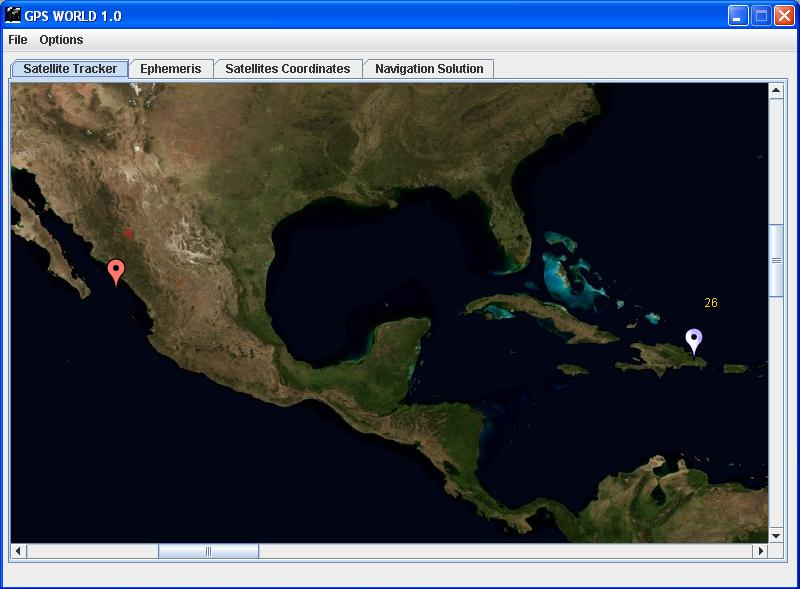Figure 15.Screen shot of the GPS WOLD GUI developed in Java

Here is a short film of one satellite moving in the map. We are updating the satellite calculation about every second.

We will not enter in detail of the implementation of the user interface in Java, but if interested in writing your own , please refer to www.sun.com, and make sure you look up the javax.comm API so that you are able to connected serially to the microcontroller. Essentially the application serves as a custom, sophisticated version of HyperTerminal.

That being said lets move on to explain how the True Anomaly θ is calculated for times after the TOE (time at which the ephemeris were sent). The problem arises with the elliptical shape of the orbit, which does not allow the True Anomaly to be calculated as trivially is if we were dealing with circular orbit (for which the True Anomaly would had been a linear function of time). The  challenge is to derive equation of motion to determine the time dependence of a satellite within its orbit. This was done in FindSat() and here we refer the reader to GPS: Theory and Practice by B. Hofmann-Wellenhof to see more of the theory as its beyond the scope of this paper to explain terms such as Mean Anomaly and Eccentric Anomaly which are used to derive the equations of motion.

Once the ephemerides and the pseudorange have been extracted for every satellite (and we know the ECEF coordinates for at least four satellites) it is time to calculate the navigation solution. The technique implemented in this project is based on the code range determined by pseudoranges. By the code range we mean the pseudorange obtained at a known time tagged as the receiver time. The pseudorange from satellite j to the receiver measured at time t is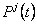. The pseudo range may be represented mathematically as: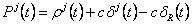, where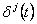is the satellite clock offset (obtained from the satellites along with the ephemerides),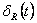is the receiver clock offset (unknown), and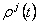is the actual range. The later is the true distance from the jth satellite at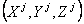to the receiver at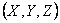, or just simply: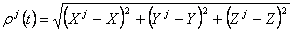. If we plug the equation for the true range into that of the pseudo range and rearrange it so that the left side has the known measured values and the four unknown values X, Y, Z,are on the right then we are left with: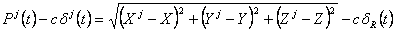.

Since we have one equation like this for every satellite that we are tracking then we only need to track four satellites to have four equations and hence solve for our four unknowns. The Newton-Raphson method which we previously explained was used to solve the equation above. We first chose an initial guess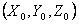which differs from the true position by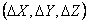. We need to calculate these differences in order to correct this initial guess as to approach the true position. We begin by performing a first-order Taylor expansion on expanding of the actual range: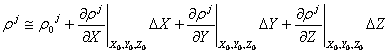, where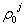is the initial guess for the actual range. This equation is then substituted into that of the pseudorange and rearranged (moving all known value to the left and leaving the unknowns on the right) to obtain: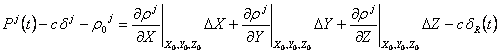,

where the derivatives (calculated at the initial guess) are given by: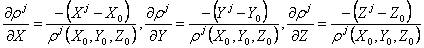. Given that we need four satellite (hence four sets of equations) to solve for the four unknowns it is most convenient to use the following matrix notation: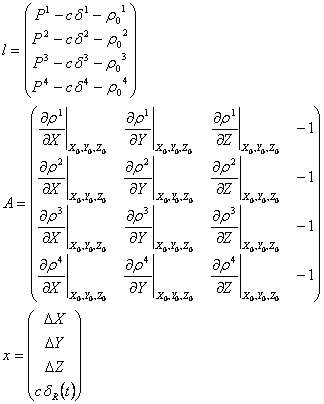This linear system has a solution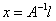which is calculated using the Gauss-Jordan Elimination method which we described earlier. The differences are then added to the initial guess to calculate de actual position. To improve the accuracy of this method it must be repeated several times, and at each point the previous actual position becomes the new guess position.

MCU Serial Communication:

To implement serial communication on the Mega32, we referred to the ECE476 serial page. Essentially all we do is set up the UART by turning on  the transmitter and receiver(UCSRB = 0b00011000). We set UBRRL to 103 to set the baud rate to 9600, which is the baud rate at which the DG14  transmits data by default. We also found convenient to turn on the receiver and transmitter at the beginning of every  iteration ( allowing to send commands to the DG14 and the Java application, and turning it off at the end of the iteration which clears the receiver buffer just in case the DG14 was sending some extra information we did not want to parse(otherwise in the next iteration the program could parse incorrectly).

The serial connections that we made with some wires and connectors recycled from old cables is shown  in Figure 16. In case the reader is wondering what is going on, the DG14 assumes that the connections specified in page 13 of the manual are going to a null modem cable.  We took a shortcut and implemented the null modem cable  between  the  DB25 and the DB9 connectorsFigure 16: DG14 Receiver Power-I/O ConnectionsTop

Results

In order to verify the accuracy of our calculations we connected our receiver to antenna #2 in the Flex Laboratory (Phillips hall, Cornell University) since we already had the exact location of this point. Our project outputted the following location: latitude = 42.4439200°, longitude = -76.482681°, and alt = 305 meters for one of many calculations of the navigation solution . These values were compared to the true position (lat = 42.444007°, lon = -76.482236°, alt = 234.597731m) to find the error (using ECEF coordinates):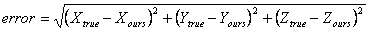,

which turned out to be approximately 123 meters.

This result was quite satisfactory given that we did not account for ionospheric corrections, and that the floating point conversion may have reduced the accuracy in the data. This result gives us a ball park estimate of the error give or take a few hundred meters. This is satisfactory because with the given complexity of the project and the many challenges that we overcome is almost a miracle that we were this close.

Conclusions

The GPS World project met all specification we set out to do when we first wrote the proposal. It tracks satellites with a very small error in their position and it calculates a three dimensional navigation solution( altitude, latitude, and longitude) within a reasonable error given the single precision floating  point  problem and the many corrections that could still be implemented in further development of the project. It is too early into the project to consider any patents, as it is difficult to compete with large companies that have been implementing receivers for many years now, nevertheless the project leaves much room for improvement and future developments.

Further Developments:

1-) Lost of debugging and testing. This being a first version it is sure to have a couple of bugs here and there. We actually recommend that before implementing the next version of GPS World to test and verify that all errors are due to hardware/software limitations( double vs single precision floating point) and not programming errors or conceptual ones, like making sure leap seconds are accounted for which we believe to have done (13 seconds), but later found out it has changed to 14 leap seconds by GPS standards.

2-)  Currently we are using four satellites ( the first four the DG14 locks on with available pseudoranges) to compute the navigation solution. Using the over-determined solution which involves using 4 or more satellites  to calculate the navigation solution is sure to improve the accuracy on most of the cases.

3-) Ionespheric corrections where not implemented and this could also improve the accuracy by a couple of meters or more.

4-) Our linear equation solver uses Gauss-Jordan Elimination method and it could be changed to one more efficient using the LU decomposition method. This may improve on the memory usage which can be a big factor when calculating the over determined solution.

6-) A more ambitious goal would be to try to develop the whole receiver now that you know the back end works. Having the Atmega32 communicating with the GP2021 should be an challenging task that by no means looks impossible to accomplish.

7-) Develop a velocity solution.

As one could see the list could keep on expanding as far as imagination allows it, and that’s great!

Intellectual Property and Legal Considerations:

Nowadays GPS technology is everywhere, and society becomes more dependent on it everyday. Right now this project serves mainly as an educational tool that should not be integrated to any other appliance that may be of value to you as there has not been enough testing.  As an educational tool we expect other students to feel free to expand on this and  encourage them to build on  and re-use our code as long as proper credit is given to the initial creators: Roberto Torralbas and José Manuel Alvarez.

What is captivating about GPS WORLD is that it’s an academic (non-for-profit) project which accomplishes all that same things that expensive commercial receiver do, by using a simple ATMega32 microcontroller, instead of a more powerful chip.  Hence, our venture does not violate on any copyright laws or patents since it constitutes a new solution to a common problem. When it comes to legal considerations is hard to find ways in which our little project could violate any. All the code was implemented from our knowledge of classes such as ECE 415 offered by Prof. Kintner at Cornell University and commonly known algorithms( Gauss-Jordan Elimination). The project currently uses the signal available to the public and not the precise code available to the military. Even though is very, very, unlikely anyone can decode the Y code we will recommend to future geniuses that try to further develop this project not to even attempt it as it could land you in jail.

Ethical Considerations:

The IEEE Code of Ethics referenced below was followed during the design and implementation of this project:

1. to accept responsibility in making decisions consistent with the safety, health and welfare of the public, and to disclose promptly factors that might endanger the public or the environment;

All decisions made during the design and implementations of this project were done responsibly and looking after the safety, health, and welfare of the public. The final product hence presents no eminent danger to the public, but will be handle with great care in case that some endangering factors arise in the future.

1. to avoid real or perceived conflicts of interest whenever possible, and to disclose them to affected parties when they do exist;

We believe that are no conflicts of interest, and hence no affected parties.

1. to be honest and realistic in stating claims or estimates based on available data;

All claims made and detailed throughout this report have been shown to both theory and practice.

1. to reject bribery in all its forms;

This is an academic non-for-profit project and no bribery issues arouse at any point during the design or implementation of it.

1. to improve the understanding of technology, its appropriate application, and potential consequences;

We hope that our project will help in the understanding of GPS theory ad application, and its many capabilities.

1. to maintain and improve our technical competence and to undertake technological tasks for others only if qualified by training or experience, or after full disclosure of pertinent limitations;

Both team members have taken introductory and advance GPS theory, implementation and receiver design courses at Cornell University. Their experience with microcontrollers in ECE 476 on the other hands has also served them well to implement all the desired project goals in a neat and timely fashion.

1. to seek, accept, and offer honest criticism of technical work, to acknowledge and correct errors, and to credit properly the contributions of others;

Critiques and suggestions by Prof. Bruce Land and the other ECE 476 labs TAs have been well received and greatly appreciated by our group. We also appreciated the help provided by Prof. Paul Kintner and (PhD) Alex Cerruti regarding GPS receiver specifics.

1. to treat fairly all persons regardless of such factors as race, religion, gender, disability, age, or national origin;

Both team members have sustain all common ethical considerations regarding each other as well as members from other teams, teaching assistants, professor, and any other personnel involved in this project.

1. to avoid injuring others, their property, reputation, or employment by false or malicious action;

Both members watched over the well-being of others and their property during the course of this project.

1. to assist colleagues and co-workers in their professional development and to support them in following this code of ethics.

Our team has and will continue to provide support to our peers in their professional and social endeavors.Top

Acknowledgment

We want to thank Prof. Paul Kintner, and  Alex Cerruti (PhD candidate) for all teaching us GPS theory with great dedication and letting us use their DG14  receiver.

Also we want to thank  Prof  Bruce Land for all his help during the course , his dedication to teaching, and letting us embark on this ambitious goal.

Appendices

Code:

• constants.h (header file with constants used)
• gps.c (CodeVision C program for programming Mega32)

Pictures: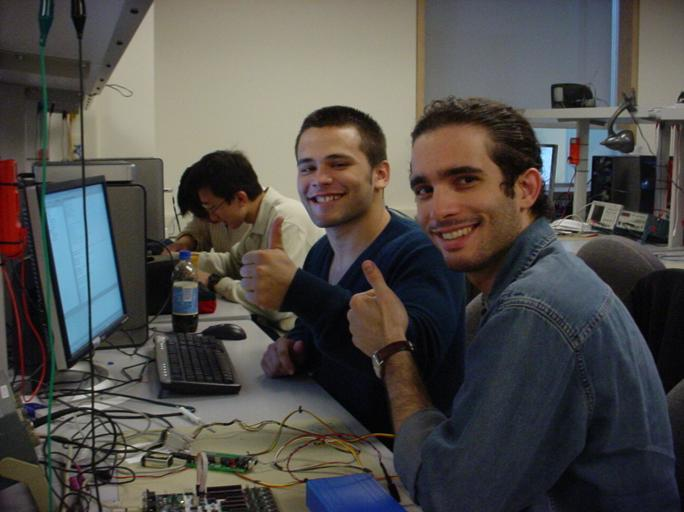Figure 17: Mission Accomplished

Parts:

 Part Cost Supplier ATMega32 \$8.00 ECE 476 Lab Custom PC board \$5.00 ECE 476 Lab ATMEL STK500 \$15.00 ECE 476 Lab THALES DG14 Receiver Free Prof. Paul Kintner ACC GPS Antenna (model GPS-P1MAM) Free Prof. Paul Kintner RS-232 Connectors (1 DB25 male and 2 DB9 female) Free Spare Parts Total \$32.00

Work Distribution:

All tasks except the Java application (Roberto Torralbas) were carried out by both team members in the ECE 476 lab and the home-made lab room we created.

References

Datasheets:

   Mega32 microcontroller

   THALES DG14

Used images:

   Blue Marble (for high resolution world map)

   Google Maps (for marker images)Top